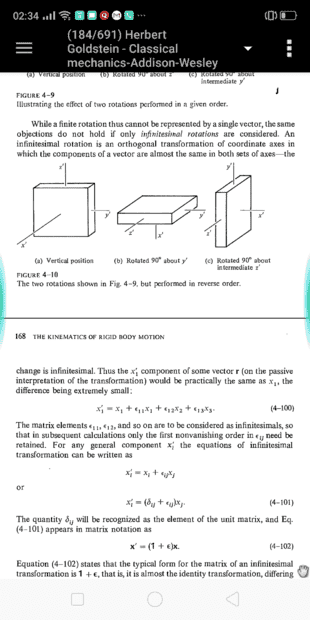# Infinitesimal rotations

• A
KashmirCan anybody please help me to understand that why under infinitesimal rotation ##x1## transforms in the way as shown in equation 4-100?

This is from Goldstein's Classical Mechanics page chapter 5 and page 168 on the Kinematics of Rigid body motion.

Last edited:

Homework Helper
Gold Member
2022 Award
https://www.physicsforums.com/attachments/292210

Can anybody please help me to understand that why under infinitesimal rotation ##x'_1## transforms in the way as shown ?

This is from Goldstein's Classical Mechanics page chapter 5 and page 168 on the Kinematics of Rigid body motion.

•Kashmir
Kashmir
Thank you. Edited.

Homework Helper
Gold Member
2022 Award
The way I would do it is to write out the rotation matrix for a rotation of ##\theta## about the ##x_1## axis. And apply this matrix to an arbitrary vector ##(x_1, x_2,x_3)##.

When ##\theta## is small, we have ##\cos \theta \approx 1##, ##\sin \theta \approx \theta##. If you apply those approximations you should get the limit for an infinitesimal rotation.

I think Goldstein is just using generic matric entries, rather than ##\cos \theta## and ##\sin \theta## explicity.

•Kashmir
Kashmir
Wouldn't it be more general to apply these trigonometric limits for small angles directly into the rotation matrix here ##A=\left[\begin{array}{ccc}\cos \psi \cos \phi-\cos \theta \sin \phi \sin \psi & \cos \psi \sin \phi+\cos \theta \cos \phi \sin \psi & \sin \psi \sin \theta \\ -\sin \psi \cos \phi-\cos \theta \sin \phi \cos \psi & -\sin \psi \sin \phi+\cos \theta \cos \phi \cos \psi & \cos \psi \cdot \sin \theta \\ \sin \theta \sin \phi & -\sin \theta \cos \phi & \cos \theta\end{array}\right]## reducing to ##A=\left[\begin{array}{ccc}1-\phi \psi & \phi+\psi & \psi \theta \\ -\psi-\phi & -\psi \phi+1 & \theta \\\theta \phi & -\theta & 1\end{array}\right]## hence the required equation A-400 ?

Homework Helper
Gold Member
2022 Award
Yes I got the idea. Thank you. Wouldn't it be more general to apply these trigonometric limits for small angles directly into the rotation matrix here ##A=\left[\begin{array}{ccc}\cos \psi \cos \phi-\cos \theta \sin \phi \sin \psi & \cos \psi \sin \phi+\cos \theta \cos \phi \sin \psi & \sin \psi \sin \theta \\ -\sin \psi \cos \phi-\cos \theta \sin \phi \cos \psi & -\sin \psi \sin \phi+\cos \theta \cos \phi \cos \psi & \cos \psi \cdot \sin \theta \\ \sin \theta \sin \phi & -\sin \theta \cos \phi & \cos \theta\end{array}\right]## reducing to ##A=\left[\begin{array}{ccc}1-\phi \psi & \phi+\psi & \phi \theta \\ 4 \beta-\phi & -\psi \phi+1 & \theta \\ -\psi & -\theta & 1\end{array}\right## hence the required equation A-400 ?
We simply want to analyse infinitesimal rotations about the three coordinate axes. Those have a well-known simple form.

Kashmir
We simply want to analyse infinitesimal rotations about the three coordinate axes. Those have a well-known simple form.
I agree. We can also think like this:
Given a general rotation matrix A defined with the three Euler angles phi, theta and psi we can find the limit by letting all three go to zero and find that the infinitesimal rotation is
##A=\left[\begin{array}{ccc}1-\phi \psi & \phi+\psi & \psi \theta \\ -\psi-\phi & -\psi \phi+1 & \theta \\\theta \phi & -\theta & 1\end{array}\right] =I+e## exactly as the author writes at equation A-102 .

Homework Helper
Gold Member
2022 Award
I agree. We can also think like this:
Given a general rotation matrix A defined with the three Euler angles phi, theta and psi we can find the limit by letting all three go to zero and find that the infinitesimal rotation is
##A=\left[\begin{array}{ccc}1-\phi \psi & \phi+\psi & \psi \theta \\ -\psi-\phi & -\psi \phi+1 & \theta \\\theta \phi & -\theta & 1\end{array}\right] =I+e## exactly as the author writes at equation A-102 .
Possibly. I don't have Goldstein, so I don't know where he's going with this. I might have misunderstood what he's trying to do.

•Kashmir
Kashmir
Possibly. I don't have Goldstein, so I don't know where he's going with this. I might have misunderstood what he's trying to do.